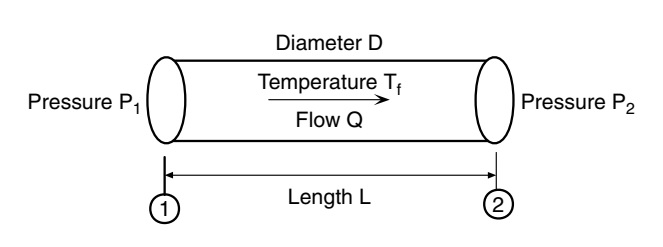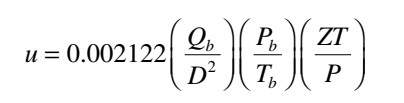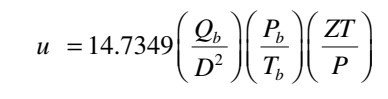# Velocity of Gas in a Pipeline

The velocity of gas flow in a pipeline represents the speed at which the gas molecules move from one point to another. Due to compressibility, the gas velocity depends upon the pressure and, hence, will vary along the pipeline even if the pipe diameter is constant. The highest velocity will be at the downstream end, where the pressure is the least. Correspondingly, the least velocity will be at the upstream end, where the pressure is higher.The gas velocity at any point of a pipeline is as follows in USCS Units:Formula for Calculating gas velocity at any point of pipeline in USCS Unit.

Where,

u=upstream gas velocity, ft/s
Qb=gas flow rate, measured at standard conditions, ft3/day (SCFD)
D=pipe inside diameter, in.
Pb=base pressure, psia
Tb=base temperature, °R(460 + °F)
P=upstream pressure, psia
T=upstream gas temperature, °R(460 + °F)
Z= gas compressibility factor at upstream conditions, dimensionless

The gas velocity at any point of a pipeline is as follows in SI Units:Formula for Calculating gas velocity at any point of pipeline in SI Unit.

Where,
u=upstream gas velocity, m/s
Qb=gas flow rate, measured at standard conditions, m3/day (SCFD)
D=pipe inside diameter, mm.
Pb = base pressure, Kpa
Tb= base temperature, °K(273 + °C)
P= upstream pressure, Kpa
T= upstream gas temperature, °K(273 + °C)
Z=
gas compressibility factor at upstream conditions, dimensionless.